• Python 运算符优先级
千次阅读
2020-11-27 21:30:32

Python 运算符优先级

目前,我们已经学习了"算术"、"条件"、"逻辑"运算符，如果，当这些运算符同时出现在同一行代码时，那么，究竟它们谁先执行呢？还是按从左往右执行呢？还是按从右往左执行呢？

所以，在接下来的时间里面，我们就要介绍一下"运算符优先级"这个内容了。

在同一行代码中，同时出现多种不同的运算符时，那么"优先级"高的运算符就会先执行，而"同级"的运算符，则按从左往右执行，"优先级"低的运算符，就最后执行。

1、 接下来，我们来看看，具体的运算符优先级如何划分

not 优先级高于 算术运算符

算术运算符 优先级高于 关系运算术

关系运算术优先级高于 "and"和"not"

"and"和"not"优先级高于 "="

通过上面的介绍，我们就可以看到各种不同的运算符之间的优先级的高低了，优先级最高就是not，而最低就是=

2、 接下来，我们再来看看同一个优先级的运算符又是如何划分执行的先后次序。

算术运算符 —— 乘法、除法、模运算 优先级高于 加法、减法

算术运算符 —— 乘法、除法、模运算 出现在同一行代码中，则按从左到右的顺序执行

算术运算符 —— 加法、减法， 出现在同一行代码中，则按从左到右的顺序执行

关系运算术——出现在同一行代码中，按从左往右顺序执行

非常好，现在，我们已经掌握了Python中的“运算符优先级”这些知识点，但是，如何去运行用它呢？所以，我们就要通过下方的按钮进入到游戏中，然后，运用“运算符优先级”去完成任务，这样，才可以加深大家对每个知识点的理解和运用，以及“尽快进入到编程的状态”。

不要停留在理论规则上面，快动起来吧！

（通过游戏模式学习与训练，需要在电脑上运行）

更多相关内容
• Python运算符优先级教程我们知道，在数学运算中，有 “先乘除后加减” 的运算规则，在我们程序语言中一样有运算符优先级问题，来决定我们运算的顺序问题，这就是运算符优先级。即所谓运算符优先级，指的是在...

Python运算符优先级教程

我们知道，在数学运算中，有 “先乘除后加减” 的运算规则，在我们程序语言中一样有运算符的优先级问题，来决定我们运算的顺序问题，这就是运算符的优先级。

即所谓运算符的优先级，指的是在含有多个运算符的式子中，到底应该先计算哪一个，后计算哪一个。

Python运算符运算规则

需要注意的是，Python 语言中大部分运算符都是从左向右执行的，只有单目运算符、赋值运算符和三目运算符例外，它们是从右向左执行的。

Python运算符中，如果有小括号，即 ()，则小括号的优先级最高。

Python运算符优先级表

运算符说明

Python运算符

优先级

小括号

()

20

索引运算符

x[index] 或 x[index:index2[:index3]]

18、19

属性访问

x.attrbute

17

乘方

**

16

按位取反

~

15

符号运算符

+（正号）或 -（负号）

14

乘、除

*、/、//、%

13

加、减

+、-

12

位移

>>、<<

11

按位与

&

10

按位异或

^

9

按位或

|

8

比较运算符

==、!=、>、>=、<、<=

7

is 运算符

is、is not

6

in 运算符

in、not in

5

逻辑非

not

4

逻辑与

and

3

逻辑或

or

2

案例

运算符优先级

乘除优先级比加减高

print("嗨客网(www.haicoder.net)")

# 乘除优先级比加减高

a = 1 + 2 * 3

b = 10 - 10 / 5

print('a =', a, 'b =', b)

程序运行后，控制台输出如下：我们首先定义了 1+2*3 的结果，因为乘法的优先级高于加法，因此这里我们先计算乘法，再计算加法，所以最终结果为 1+6=7。

接着我们定义变量 b，其值为表达式 10 - 10 / 5 的结果，因为除法的优先级高于减法，因此这里我们先计算除法，再计算减法，所以最终结果为 10-2=8。

小括号改变运算符优先级

小括号的运算符优先级最高

print("嗨客网(www.haicoder.net)")

# 小括号的运算符优先级最高

a = (1 + 2) * 3

b = (10 - 2) / 4

print('a =', a, 'b =', b)

程序运行后，控制台输出如下：我们首先定义了变量 a，其值为表达式 (1 + 2) * 3 的结果，因为小括号的运算符优先级最高，因此这里我们先计算小括号里面的加法，再计算乘法，所以最终结果为 3*3=9。

接着我们定义变量 b，其值为表达式 (10 - 2) / 4 的结果，因为小括号的运算符优先级最高，因此这里我们先计算减法，再计算除法，所以最终结果为 8 / 4 = 2。

Python运算符优先级总结

即所谓运算符的优先级，指的是在含有多个逻辑运算符的式子中，到底应该先计算哪一个，后计算哪一个。

Python 中运算符的运算规则是，优先级高的运算符先执行，优先级低的运算符后执行，同一优先级的运算符按照从左到右的顺序进行。

Python运算符中，如果有小括号，即 ()，则小括号的优先级最高。

展开全文• 分享哪位大神帮忙解释一下print(3==3>2)print((3==3)>...2)，两部分都是True，所以最终结果也是True 另外两个有括号，括号的优先级高，所以先计算括号里面的内容，然后再进行比较。 print (...

分享哪位大神帮忙解释一下print(3==3>2)print((3==3)>2)print(3==(3>2))记忆像一把利刃划开时间的屏障，回到那些最嚣张跋扈的年华。

print(3==3>2) 等价于(3==3) and (3>2)，两部分都是True，所以最终结果也是True 另外两个有括号，括号的优先级高，所以先计算括号里面的内容，然后再进行比较。 print ((3==3)>2)等价print(True>2)，最终False print(3==(3>2))等价print(3==True世上最痛苦的事，不是生老病死，而是生命的旅程虽短，却充斥着永恒的孤寂。

python 操作符**与*的用法

请问**与*是什么意思，能用文字加代码讲解下吗？非常我并没有让别人快乐的能力，每次都是这样暗示着，所以也一点都不怪对方总是三分热情。甚至松了一口气，要是他误认为我真的是一个活泼女孩儿怎么办。

**两个乘号就是乘方，比如2**4,结果就是2的4次方，结果是16。 代码样例： 想要摘星星的孩子，孤独是我们的必修课，我不怕自己努力了不优秀，我只怕比我优秀的人比我更努力。

*如果是字符串、列表、元组与一个整数N相乘。

python逻辑运算符有哪些以前坐公交你总是搂着我怕我摔，现在一个人坐公交才发现那些一起经过的站点竟如此漫长。

1. 比较运算符： 如果比较式成立，返回True；不成立，则返回False。 常见的比较运算符如下： 除了简单的两值相比之外，比较运算符还支持比较复杂的比较。 (1)多条件比较： 可以先给变量a赋值，然后做多条件比较。 1 a = 3 2 print(1 < a < 5) 你将我高高的举起，又将我重重的摔在了地上，你真善良。

python 中的0.3==0.3*1 与 0.3==3*0.1 比较运算符因为计算机的浮点表示本来就不精准。

python 操作符“**”与“*”是什么意思？

python中位运算符<

>>> 10 >> 10 >> 15>>>是按位右移。

Python运算符优先级规则有哪些

以下运算符优先级顺序依次递增：Lambda #运算优先级最低逻辑运算符: or逻辑运算符: and逻辑运算符:not成员测试: in, not in同一性测试: is, is not比较: =,。=,==按位或: |按位异或: ^按位与: &移位: >加法与减法: + ,-乘法、除法与取余: *, / ,%真怀恋小时候啊，天热的时候我也可以像男人一样光着膀子了。

python用条件运算符的嵌套完成此题学习成绩>=90分level = 'A' if point >= 90 else ('C' if point < 60 else 'B')有时候总感觉别人忽略了自己，想想可能是自己太闲了。

python的is和is not运算符是干什么用的思念是一种幸福的忧伤，是一种甜蜜的惆怅，是一种温馨的痛苦。

简单来说，python中的is与is not运算符可判断两个对象是否为同一对象。 若为同一个对象，则对象1 is 对象2为True 。 反正，若非同一个对象，则对象1 is not 对象2为True 要理解Python中的is和is not运算符，首先需要知道Python中对象包含的三个有时候，适合你的那个人其实一直在那儿，只是那些错的人挡住了你的视线，你才没有看见。

展开全文• 一、Python超级计算器 1.运算符 Python算数运算符 以下假设变量：a = 10 ，b = 20

# 一、Python算数运算符

以下假设变量：a = 10 ，b = 20

运算符描述实例
+加（两个对象相加）a+b输出结果为30
-减（得到负数或是一个数减去另一个数）a-b输出结果为-10
*乘（两个数相乘或是返回一个被重复若干次的字符串）a*b输出结果为200
/除（x除以y）b/a输出结果为2
%取模（返回除法的余数）b%a输出结果为0
**幂（返回x的y次幂）a**b为10的20次方
//取整除（返回商的整数部分-向下取整）9//2 输出结果为4 -9//4 输出结果为-5

下面是Python代码：

a = 21
b = 10
c = 0

c = a + b
print("1 - c 的值为：", c)

c = a - b
print("2 - c 的值为：", c)

c = a * b
print("3 - c 的值为：", c)

c = a / b
print("4 - c 的值为：", c)

c = a % b
print("5 - c 的值为：", c)

# 修改变量 a 、b 、c
a = 2
b = 3
c = a**b
print("6 - c 的值为：", c)

a = 10
b = 5
c = a//b
print("7 - c 的值为：", c)


运行结果：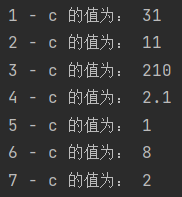# 二、Python比较运算符

以下假设变量：a = 10 ，b = 20

运算符描述实例
==等于（比较两个对象是否相等）(a == b)返回False
!=不等于（比较两个对象是否不相等）(a != b)返回True
>大于（返回x是否大于y）(a > b)返回False
<小于（返回x是否小于y）(a < b)返回True
>=大于等于（返回x是否大于等于y）(a >= b)返回False
<=小于等于（返回x是否小于等于y）(a <= b)返回True

所有比较运算符返回 1 表示真，返回 0 表示假。
这分别与特殊的变量 True 和 False 等价（注意这些变量名的大小写）。

下面是Python代码：

a = 21
b = 10
c = 0

if ( a == b ):
print ("1 - a 等于 b")
else:
print ("1 - a 不等于 b")

if ( a != b ):
print ("2 - a 不等于 b")
else:
print ("2 - a 等于 b")

if ( a < b ):
print ("3 - a 小于 b")
else:
print ("3 - a 大于等于 b")

if ( a > b ):
print ("4 - a 大于 b")
else:
print ("4 - a 小于等于 b")

# 修改变量 a 和 b 的值
a = 5;
b = 20;
if ( a <= b ):
print ("5 - a 小于等于 b")
else:
print ("5 - a 大于  b")

if ( b >= a ):
print ("6 - b 大于等于 a")
else:
print ("6 - b 小于 a")


运行结果：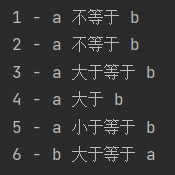# 三、Python赋值运算符

以下假设变量：a = 10 ，b = 20

运算符描述实例
=简单的赋值运算符c = a + b 将 a + b 的运算结果赋值给c
+=加法赋值运算符c += a 等效于 c = c + a
-=减法赋值运算符c -= a 等效于 c = c - a
*=乘法赋值运算符c *= a 等效于 c = c * a
/=除法赋值运算符c /= a 等效于 c = c / a
%=取模赋值运算符c %= a 等效于 c = c % a
**=幂赋值运算符c **= a 等效于 c = c ** a
//=取整赋值运算符c //= a 等效于 c = c // a

下面是Python代码：

a = 21
b = 10
c = 0

c = a + b
print ("1 - c 的值为：", c)

c += a
print ("2 - c 的值为：", c)

c *= a
print ("3 - c 的值为：", c)

c /= a
print ("4 - c 的值为：", c)

c = 2
c %= a
print ("5 - c 的值为：", c)

c **= a
print ("6 - c 的值为：", c)

c //= a
print ("7 - c 的值为：", c)


运行结果：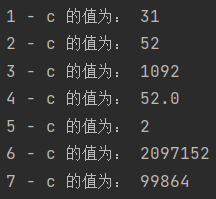# 四、Python位运算符

按位运算符是把数字看做二进制来进行计算的。

a = 60，b = 13其二进制格式如下：
a = 0011 1100
b = 0000 1101

运算符描述实例
&按位与运算符：参与运算的两个值，如果两个相应位都为1，则该位的结果为1，否则为0(a & b)输出结果为 12 ，二进制解释：0000 1100
|按位或运算符：参与运算的两个值，只要对应的两个二进位有一个为1，结果位就为1(a | b)输出结果为 61 ，二进制解释：0011 1101
^按位异或运算符：当两对应的二进位相异时，结果为1(a ^ b)输出结果为 49 ，二进制解释：0011 0001
~按位取反运算符：对数据的每个二进制位取反，即把1变为0，把0变为1。 ~x 类似于 -x-1(~a)输出结果为 -61 ，二进制解释：1100 0011
<<左移动运算符：运算数的各二进位全部左移若干位，由“<<”右边的数指定移动的位数，高位丢弃，低位补0a << 2 输出结果为 240 ，二进制解释：1111 0000
>>右移动运算符：把“>>”左边的运算数的各二进位全部右移若干位，“>>”右边的数指定移动的位数a >> 2 输出结果为 15 ，二进制解释：0000 1111

下面是Python代码：

a = 60            # 60 = 0011 1100
b = 13            # 13 = 0000 1101
c = 0

c = a & b;        # 12 = 0000 1100
print ("1 - c 的值为：", c)

c = a | b;        # 61 = 0011 1101
print ("2 - c 的值为：", c)

c = a ^ b;        # 49 = 0011 0001
print ("3 - c 的值为：", c)

c = ~a;           # -61 = 1100 0011
print ("4 - c 的值为：", c)

c = a << 2;       # 240 = 1111 0000
print ("5 - c 的值为：", c)

c = a >> 2;       # 15 = 0000 1111
print ("6 - c 的值为：", c)


运行结果：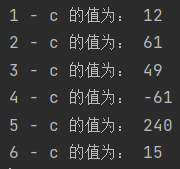# 五、Python逻辑运算符

以下假设变量：a = 10 ，b = 20

运算符逻辑表达式描述实例
andx and y布尔“与”（如果 x 为 False ，x and y 返回 False ，否则返回 y 的计算值）(a and b)返回20
orx or y布尔“或”（如果 x 是 True ，则返回 x 的值，否则返回 y 的计算值）(a or b)返回10
notnot x布尔“非”（如果 x 为 True ，则返回 False 。如果 x 为 False ，则返回 True ）not(a and b)返回 False

下面是Python代码：

a = 10
b = 20

if ( a and b ):
print ("1 - 变量 a 和 b 都为 true")
else:
print ("1 - 变量 a 和 b 有一个不为 true")

if ( a or b ):
print ("2 - 变量 a 和 b 都为 true，或其中一个变量为 true")
else:
print ("2 - 变量 a 和 b 都不为 true")

# 修改变量 a 的值
a = 0
if ( a and b ):
print ("3 - 变量 a 和 b 都为 true")
else:
print ("3 - 变量 a 和 b 有一个不为 true")

if ( a or b ):
print ("4 - 变量 a 和 b 都为 true，或其中一个变量为 true")
else:
print ("4 - 变量 a 和 b 都不为 true")

if not( a and b ):
print ("5 - 变量 a 和 b 都为 false，或其中一个变量为 false")
else:
print ("5 - 变量 a 和 b 都为 true")


运行结果：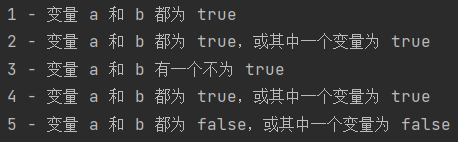# 六、Python成员运算符

运算符描述实例
in如果在指定的序列中找到值返回 True ，否则返回 Falsex 在序列 y 中，如果 x 在 y 序列中返回 True
not in如果在指定的序列中没有找到值返回 True ，否则返回 Falsex 不在序列 y 中，如果 x 不在 y 序列中返回 True

下面是Python代码：

a = 10
b = 20
list = [1, 2, 3, 4, 5 ];

if ( a in list ):
print ("1 - 变量 a 在给定的列表中 list 中")
else:
print ("1 - 变量 a 不在给定的列表中 list 中")

if ( b not in list ):
print ("2 - 变量 b 不在给定的列表中 list 中")
else:
print ("2 - 变量 b 在给定的列表中 list 中")

# 修改变量 a 的值
a = 2
if ( a in list ):
print ("3 - 变量 a 在给定的列表中 list 中")
else:
print ("3 - 变量 a 不在给定的列表中 list 中")


运行结果：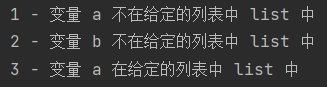# 七、Python身份运算符

身份运算符用于比较两个对象的存储单元

运算符描述实例
isis 是判断两个标识符是不是引用自一个对象x is y ，类似 id(x) == id(y) ，如果引用的是同一个对象，则返回 True ，否则返回 False
is notis not 是判断两个标识符是不是引用自不同对象x is not y ，类似 id(x) != id(y) ，如果引用的不是同一个对象，则返回 True ，否则返回 False

下面是Python代码：

a = 20
b = 20

if ( a is b ):
print ("1 - a 和 b 有相同的标识")
else:
print ("1 - a 和 b 没有相同的标识")

if ( id(a) == id(b) ):
print ("2 - a 和 b 有相同的标识")
else:
print ("2 - a 和 b 没有相同的标识")

# 修改变量 b 的值
b = 30
if ( a is b ):
print ("3 - a 和 b 有相同的标识")
else:
print ("3 - a 和 b 没有相同的标识")

if ( a is not b ):
print ("4 - a 和 b 没有相同的标识")
else:
print ("4 - a 和 b 有相同的标识")


运行结果：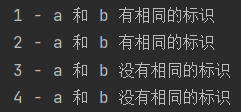# 八、Python运算符的优先级

运算符描述
**指数（最高优先级）
~ + -按位翻转，一元加号和减号（最后两个的方法名为 +@ 和 -@）
* / % //乘、除、取模和取整数
+ -加法 减法
>> <<右移、左移运算符
&位’AND’
^ |位运算符
<= < > >=比较运算符
<> == !=等于运算符
= %= /= //= -= += *= **=赋值运算符
is is not身份运算符
in not in成员运算符
not and or逻辑运算符
展开全文开发语言 后端
• python运算符包括算术运算符、赋值运算符、比较运算符、布尔运算符、位运算符。 算术运算符：+，-，*，/，//（整除），%（取余），**（幂运算）。 # 注意，一正一负时计算结果会不同 print(4%3) # 1，取余 print...
• Python 中运算符优先级，括号的优先级最高的，无论任何时候优先计算括号里面的内容，逻辑运算符优先级最低。算术运算符可以分为四种，幂运算最高，其次是正负号，然后是 “* /// %”，最后才是加减 “+ -”。...
• 1、常用运算符 （1）算术运算符 ①标准算是运算符 ②取余运算符 ③幂运算符 （2）赋值运算符 （3）比较运算符 （4）布尔运算符 （5）位运算符 2、算术运算符 （1）标准运算符：加（+）、减（-）、乘（*）...
• 种类运算优先级方法名及参数可重载 算术运算符+3__add__(self,rhs) -3__sub__(self,rhs) *233 /233 %233 **033 //333 比较运算符 赋值运算符 逻辑运算符运算符 成员运算符 ...开发语言 后端
• ## Python——运算符及其优先级

万次阅读 多人点赞 2018-08-02 16:58:17
Python 中，支持以下类型的运算符： 算术运算符 比较（关系）运算符 逻辑（布尔）运算符运算符 赋值运算符 成员运算符 身份运算符 1. 算术运算符 运算符 含义 示例 + 加：两个对象相加，...
• ## Python运算符及优先级总结

万次阅读 多人点赞 2018-08-26 13:34:02
运算符优先级 优先级 运算符 描述  最高 ** 指数 (最高优先级)   ~ + - 按位翻转, 一元加号和减号 (最后两个的方法名为 +@ 和 -@) * / % // 乘，除，取模和取整除 + - 加法减法 ...
• Python里的运算符主要有算术运算符、比较运算符、赋值运算符、位运算符、逻辑运算符、成员运算符、身份运算符这七类，而这篇文章就超超超详细地介绍了每一种运算符的用法，并给出了运算符之间的优先级
• ## Python运算符优先级

千次阅读 2019-10-15 08:25:44
Python 中运算符的运算规则是，优先级高的运算符先执行，优先级低的运算符后执行，同一优先级运算符按照从左到右的顺序进行。需要注意的是，Python 语言中大部分运算符都是从左向右执行的，只有单目运算符（例如 ...
• 网上都说比较（,<,>,>=)的优先级高于等于（==,!=,）的优先级 请问下面的代码怎么解释  >>> 3==3>2 True >>> (3==3)>2 False >>> 3==(3>2) False  我唯一能想到的就是它进行了与运算： ` 3==2 ...
• 运算符优先级 表达式是有优先级的，例如表达式："0*1+2"和"0+1*2"结果一定不一样，在"0+1*2"中优先运算"1*2" 从高到低 ​开发语言 后端
• Python 运算符_python 运算符重载_python 逻辑运算符_python 运算符优先级什么是运算符？本章节主要说明Python运算符。举个简单的例子 4 +5 = 9 。 例子中，4 和 5 被称为操作数，”+” 称为运算符Python语言...
• 一、算数运算符的介绍算数运算符运算符的一种，也称为数学运算符，是完成基本的算术运算使用的符号，用来处理四则运算。运算符描述实例+加10 + 20 = 30-减10 - 20 = -10*乘10 * 20 = 200/除10 / 20 = 0.5//取整除...
• 所有的数学运算都是从左向右进行的，Python 语言中的大部分运算符也是从左向右结合的，只有单目运算符、赋值运算符和三目运算符例外，它们是从右向左结合的，也就是说，它们是从右向左运算的。 乘法和加法是两个...程序员 编程语言
• Python 条件语句 Python条件语句是通过一条或多条语句的执行结果（True或者False）来决定执行的代码块。 可以通过下图来简单了解条件语句的执行过程: Python程序语言指定任何非0和非空（null）值为true，0 或者 ...
• I know that the statement 3 > 4 or (2 < 3 and 9 > 10) should obviously evaluate to false but I am not quite sure how python would read 4 > 5 or 3 < 4 and 9 > 8 解决方案 Comparisons are executed ...
• 逻辑运算符： not、and、or Python 3.7.3 (v3.7.3:ef4ec6ed12, Mar 25 2019, 22:22:05) [MSC v.1916 64 bit (AMD64)] on win32 True or False and True True # True or (False and True) False or not False True # ...
• 【单选题】借贷记账法的发生额试算平衡是指 【填空题】在算术运算符、关系运算符、逻辑运算符和赋值运算符中,运算优先级最高运算符是 ,最低的运算符 。 (提示:填写以上上种运算符的符合条件的中文名称) 【多选题...
• 遇到的问题今天做题的时候，碰到一个关于逻辑运算符优先级的问题，看到之后我懵了0 and 12 and 30 or 12 or 3上面的返回值是什么？我一开始以为返回的都是左边的值，但是结果和我想的不同，结果如下：In : 0 and ...
• Python运算符优先级问题作者:PHPYuan 时间:2018-07-09 23:40:42关注我，让代码变得有趣今天跟大家介绍一下Python中的常用操作符，Python中的操作符非常多有算术操作符，比较操作符，逻辑操作符等。操作符在我们的...
• 运算符优先级来确定条件的表达式中的分组。...这里，具有最高优先级运算符出现在表格上方，那些最低的显示在底部。在一个表达式，更高的优先级运算符将首先计算。例如：试试下面的例子来理解pyt...
• 一、Python中的运算符包括：算术运算符赋值运算符比较运算符布尔运算符运算符其中算术运算符包含：标准算术运算符，取余运算符（%）、幂运算符（**）标准运算符包括：加（+）、减（-）、乘（*）、/、整除（//）1、...
• 在之前的知识点中我们已经了解各种类型的Python运算符，正如四则运算有其优先级规则，Python的各类运算符之间也同样有优先级的排序。 以下表格列出了从最高到最低优先级的所有运算符运算符 描述 ** 指数 (最高...开发语言 后端
• 本节教程将讲解 Python 运算符优先级和关联性，在这之前你应该理解了 Python 运算符。对于表达式，Python 解释器将处理优先级更高的运算符，并且除了指数运算符(**)外，几乎所有运算符的处理方式都从左到右执行。...
• 算术运算符 比较（关系）运算符 赋值运算符 海象运算符 的作用是给n进行赋值，同时还可以用赋值后的n与10进行比较 逻辑运算符运算符 成员运算符 身份运算符 运算符优先级
•开发语言 后端...